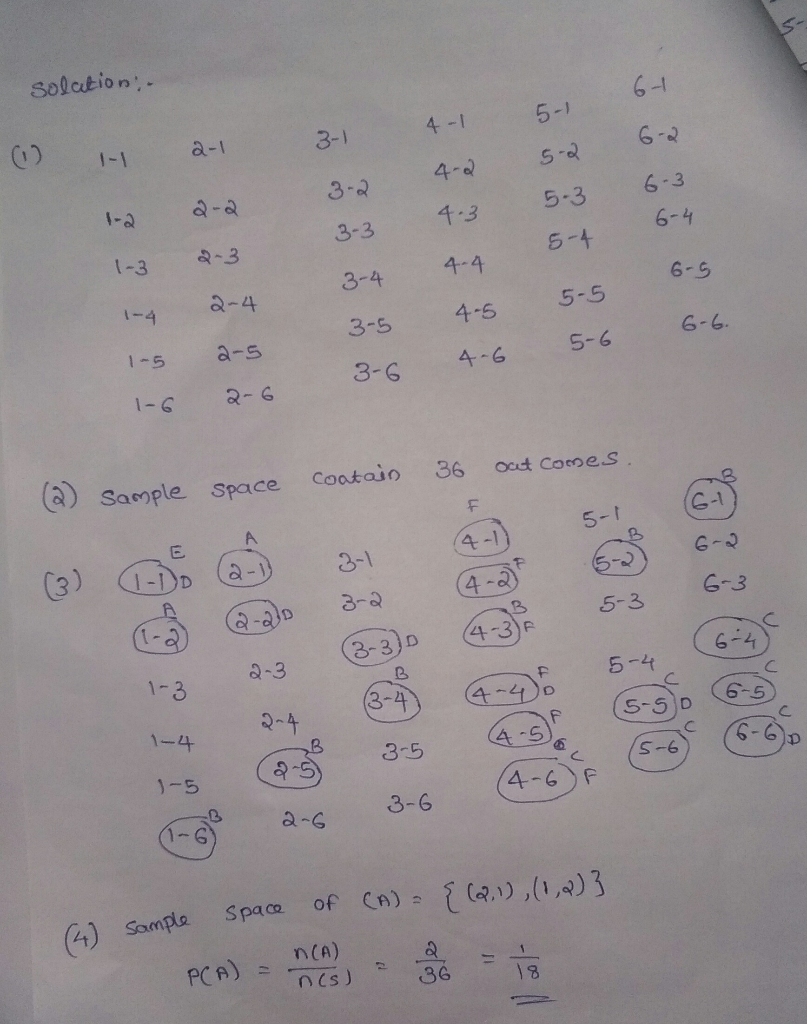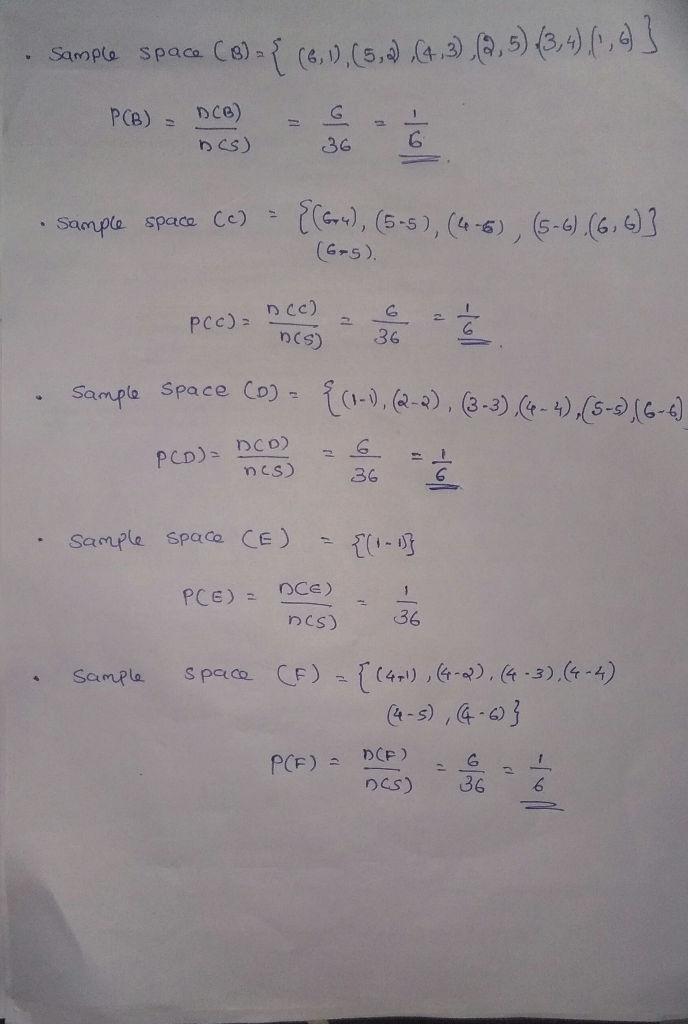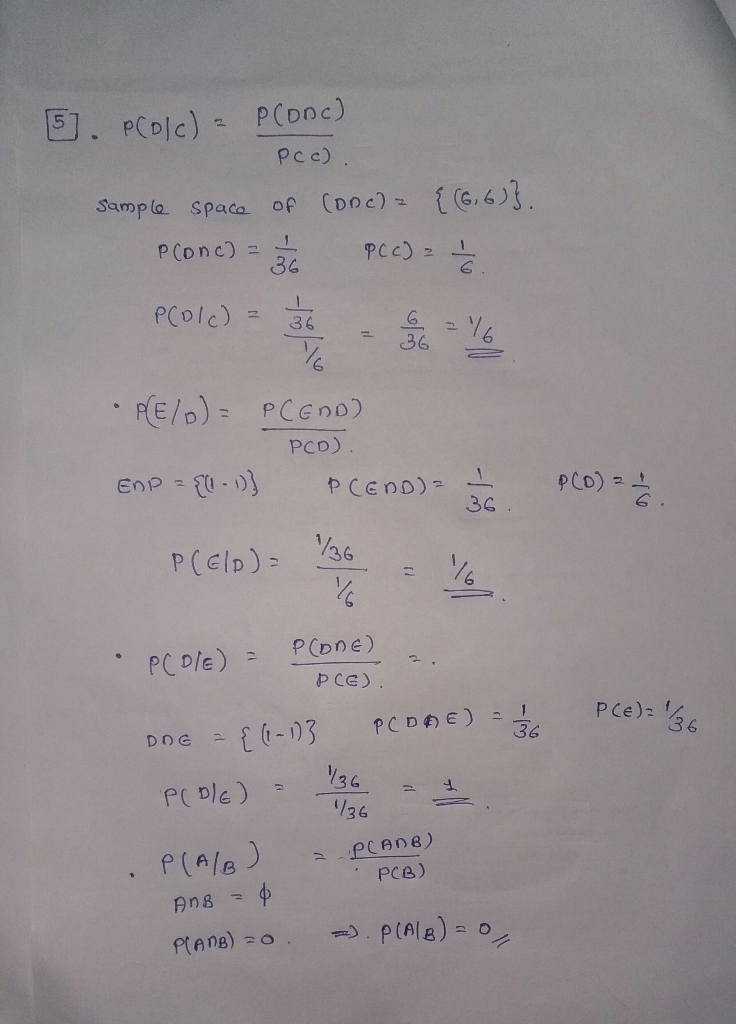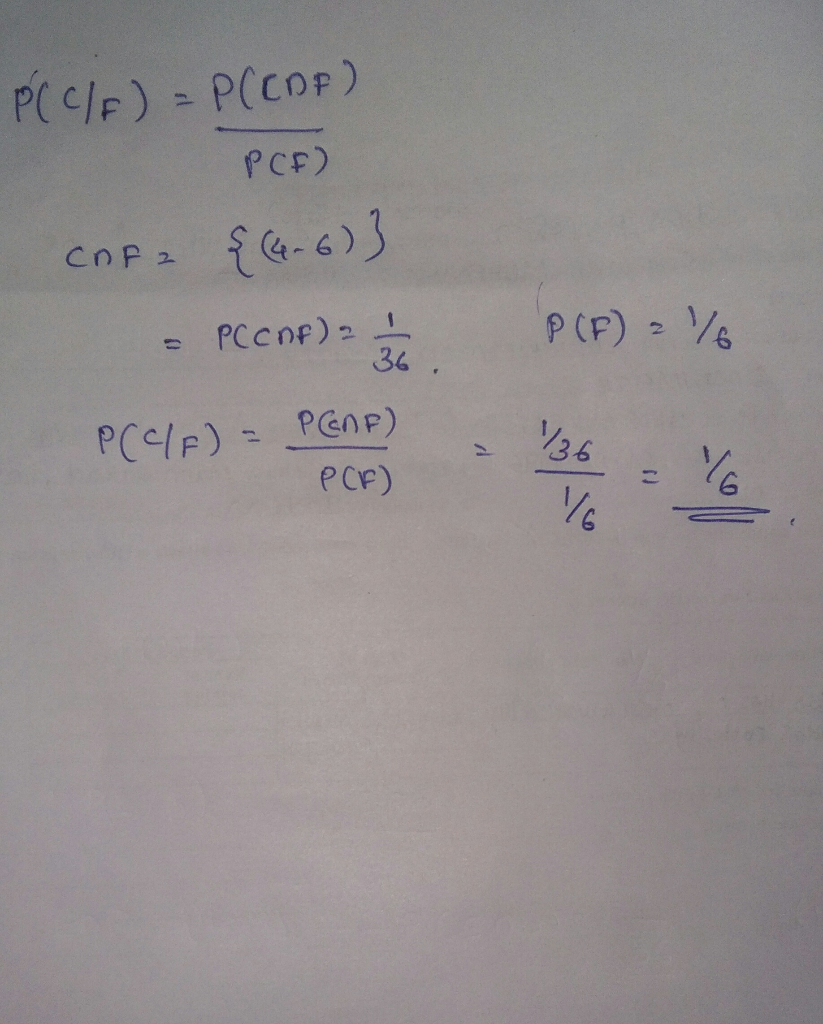##### Question

In: Statistics and Probability

# I. Consider the random experiment of rolling a pair of dice. Note: Write ALL probabilities as...

I. Consider the random experiment of rolling a pair of dice. Note: Write ALL probabilities as reduced fractions or whole numbers (no decimals).

1) One possible outcome of this experiment is 5-2 (the first die comes up 5 and the second die comes up 2). Write out the rest of the sample space for this experiment below by completing the pattern:

 1-1 2-1 1-2 1-3 1-4 1-5 1-6

2) How many outcomes does the sample space contain? _____________

3) Draw a circle (or shape) around each of the following events (like you would to circle a word in a word search puzzle). Label each event in the sample space with the corresponding letter. Event A has been done for you.

A: Roll a sum of 3.
B: Roll a sum of 7.
C: Roll a sum of at least 10.

D: Roll doubles.
E: Roll snake eyes (two 1’s). F: First die is a 4.

4) Find the following probabilities:
P(A) = _________ P(B) = _________ P(C) = _________

P(D) = _________ P(E) = _________ P(F) = _________

5) The conditional probability of B given A, denoted by P(B|A), is the probability that B will occur when A has already occurred. Use the sample space above (not a special rule) to find the following conditional probabilities:

P(D|C) = _________ P(E|D) = _________ P(D|E) = _________ P(A|B) = _________ P(C|F) = _________

6) Two events are mutually exclusive if they have no outcomes in common, so they cannot both occur at the same time.

Are C and E mutually exclusive? ___________
Find the probability of rolling a sum of at least 10 and snake eyes on the same roll, using the

sample space (not a special rule).
P(C and E) = __________

Find the probability of rolling a sum of at least 10 or snake eyes, using the sample space. P(C or E) = __________

7) Special case of Addition Rule: If A and B are mutually exclusive events, then P(A or B) = P(A) + P(B)

P(C or E) = P(C) + P(E) = ________ + ________ = _________

8) Are C and F mutually exclusive? __________ Using sample space, P(C or F) = _________ 9) Find the probability of rolling a “4” on the first die and getting a sum of 10 or more, using the

sample space.
P (C and F) = ________

10) General case of Addition Rule: P(A or B) = P(A) + P(B) – P(A and B) Use this rule to verify your last answer in #8:

P(C or F) = P(C) + P(F) – P(C and F) = ________ + ________ − ________ = _________

11) Two events are independent if the occurrence of one does not influence the probability of the other occurring. In other words, A and B are independent if P(A|B) = P(A) or if P(B|A) = P(B).

Compare P(D|C) to P(D), using the sample space: P(D|C) = ________ . P(D) = ________ .
Are D and C independent? _________
When a gambler rolls at least 10, is she more or less likely to roll doubles than usual? ___________ Compare P(C|F) to P(C), using the sample space: P(C|F) = ________ . P(C) = ________ .

Are C and F independent? __________
12) Special case of Multiplication Rule: If A and B are independent, then P(A and B) = P(A) · P(B).

P(C and F) = P(C) • P(F) = ________ · ________ = ________ .

13) Find the probability of rolling a sum of at least 10 and getting doubles, using the sample space. P(C and D) = ________ .

14) General case of Multiplication Rule: P(A and B) = P(A) · P(B|A). Use this rule to verify your answer to #13:

P(C and D) = P(C) • P(D|C) = ________ · ________ = ________ .

## Solutions

##### Expert Solution## Related Solutions

##### I. Consider the random experiment of rolling a pair of dice. Note: Write ALL probabilities as...
I. Consider the random experiment of rolling a pair of dice. Note: Write ALL probabilities as reduced fractions or whole numbers (no decimals). 1-1 2-1 3-1 4-1 5-1 6-1 1-2 2-2 3-2 4-2 5-2 6-2 1-3 2-3 3-3 4-3 5-3 6-3 1-4 2-4 3-4 4-4 5-4 6-4 1-5 2-5 3-5 4-5 5-5 6-5 1-6 2-6 3-6 4-6 5-6 6-6 2) How many outcomes does the sample space contain? _____36________ 3)Draw a circle (or shape) around each of the following events...
##### Please answer form 6-14 I. Consider the random experiment of rolling a pair of dice. Note:...
Please answer form 6-14 I. Consider the random experiment of rolling a pair of dice. Note: Write ALL probabilities as reduced fractions or whole numbers (no decimals). 1) One possible outcome of this experiment is 5-2 (the first die comes up 5 and the second die comes up 2). Write out the rest of the sample space for this experiment below by completing the pattern: 1-1 2-1 1-2 1-3 1-4 1-5 1-6 2) How many outcomes does the sample space...
##### probabilities Find the probabilities for each event. Consider rolling a pair of fair dice two times....
probabilities Find the probabilities for each event. Consider rolling a pair of fair dice two times. Let A be the total on the up-faces for the first roll and let B be the total on the up-faces for the second roll. A = {2 on the first roll}, B = {8 or more on the first roll}. A = {2 on the first roll}, B = {8 or more on the second roll}. A = {5 or less on the...
##### Consider a random experiment of rolling 2 dice. What is probability of rolling a sum larger...
Consider a random experiment of rolling 2 dice. What is probability of rolling a sum larger than 9? Select the best answer. A. 0.5 B. 0.1667 C. 0.2333 D. None of the above
##### for the experiment of rolling an ordinary pair of dice, find the probability that the sum...
for the experiment of rolling an ordinary pair of dice, find the probability that the sum will be even or a multiple of 6. ( you may want to use a table showing the sum for each of the 36 equally likely outcomes.)
##### Consider rolling two dice and let (X, Y) be the random variable pair defined such that...
Consider rolling two dice and let (X, Y) be the random variable pair defined such that X is the sum of the rolls and Y is the maximum of the rolls. Find the following: (1) E[X/Y] (2) P(X > Y ) (3) P(X = 7) (4) P(Y ≤ 4) (5) P(X = 7, Y = 4)
##### An experiment is rolling two fair dice and adding the spots together. Find the following probabilities;...
An experiment is rolling two fair dice and adding the spots together. Find the following probabilities; enter all answers as simplified fractions using the / bar between numerator and denominator, with no extra space Blank #1: Find the probability of getting a sum of 3. Blank #2: Find the probability of getting the first die as a 4. Blank #3: Find the probability of getting a sum of 8. Blank #4: Find the probability of getting a sum of 3...
##### Consider rolling a fair dice. You keep rolling the dice until you see all of the...
Consider rolling a fair dice. You keep rolling the dice until you see all of the faces (from number 1 to 6) at least once. What is your expected number of rolls?
##### Consider the experiment of rolling two dice and the following events:     A: 'The sum of the...
Consider the experiment of rolling two dice and the following events:     A: 'The sum of the dice is 8' and  B: 'The first die is an odd number' and C:  "The difference (absolute value) of the dice is 2" Find  (a)  p(A and B) (HINT: You cannot assume these are independent events.)        (b)  p(A or B)         (c)  Are A and B mutually exclusive events? Explain. (d)   Are A and B independent events? Explain. (e)   Are B and C independent events? Explain.
##### Consider the game consisting of rolling a pair of fair 6-sided dice and recording the sum....
Consider the game consisting of rolling a pair of fair 6-sided dice and recording the sum. It will cost you $1 to play the game. If the sumis less than 5, then you will win$3. However, you do not get your $1 back so your profit is$2. If you roll a sum of exactly8, thenyou will win $4. However, you do not get your$1 back so your profit is $3. Otherwise, you lose your$1. A.What is...